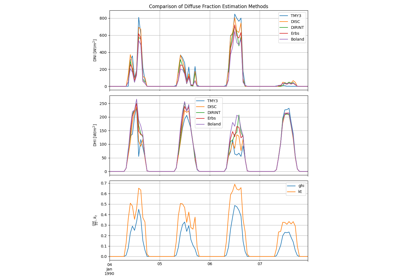pvlib.irradiance.boland(ghi, solar_zenith, datetime_or_doy, a_coeff=8.645, b_coeff=0.613, min_cos_zenith=0.065, max_zenith=87)[source]#

Estimate DNI and DHI from GHI using the Boland clearness index model.

The Boland model 1, 2 estimates the diffuse fraction, DF, from global horizontal irradiance, GHI, through an empirical relationship between DF and the clearness index, $$k_t$$, the ratio of GHI to horizontal extraterrestrial irradiance.

$\mathit{DF} = \frac{1}{1 + \exp\left(a \left(k_t - b\right)\right)}$
Parameters
• ghi (numeric) – Global horizontal irradiance. [W/m^2]

• solar_zenith (numeric) – True (not refraction-corrected) zenith angles in decimal degrees.

• datetime_or_doy (numeric, pandas.DatetimeIndex) – Day of year or array of days of year e.g. pd.DatetimeIndex.dayofyear, or pd.DatetimeIndex.

• a_coeff (float, default 8.645) – Logistic curve fit coefficient.

• b_coeff (float, default 0.613) – Logistic curve fit coefficient.

• min_cos_zenith (numeric, default 0.065) – Minimum value of cos(zenith) to allow when calculating global clearness index $$k_t$$. Equivalent to zenith = 86.273 degrees.

• max_zenith (numeric, default 87) – Maximum value of zenith to allow in DNI calculation. DNI will be set to 0 for times with zenith values greater than max_zenith.

Returns

data (OrderedDict or DataFrame) –

Contains the following keys/columns:

• dni: the modeled direct normal irradiance in W/m^2.

• dhi: the modeled diffuse horizontal irradiance in W/m^2.

• kt: Ratio of global to extraterrestrial irradiance on a horizontal plane.

References

1

J. Boland, B. Ridley (2008) Models of Diffuse Solar Fraction. In: Badescu V. (eds) Modeling Solar Radiation at the Earth’s Surface. Springer, Berlin, Heidelberg. DOI: 10.1007/978-3-540-77455-6_8

2(1,2)

John Boland, Lynne Scott, and Mark Luther, Modelling the diffuse fraction of global solar radiation on a horizontal surface, Environmetrics 12(2), pp 103-116, 2001, DOI: 10.1002/1099-095X(200103)12:2%3C103::AID-ENV447%3E3.0.CO;2-2

Notes

Boland diffuse fraction differs from other decomposition algorithms by use of a logistic function to fit the entire range of clearness index, $$k_t$$. Parameters a_coeff and b_coeff are reported in 2 for different time intervals:

• 15-minute: a = 8.645 and b = 0.613

• 1-hour: a = 7.997 and b = 0.586

## Examples using pvlib.irradiance.boland#Diffuse Fraction Estimation

Diffuse Fraction Estimation